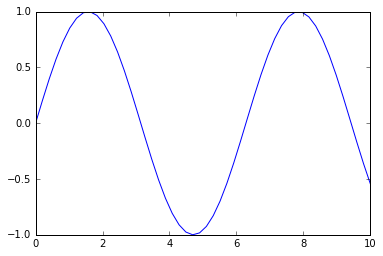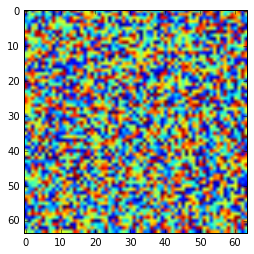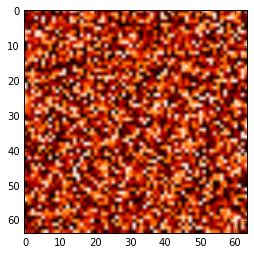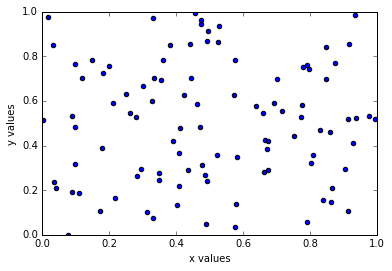# Introduction to Matplotlib¶

Now that we can start doing serious numerical analysis with Numpy arrays, we also reach the stage where we can no longer print out hundreds or thousands of values, so we need to be able to make plots to show the results.

The Matplotlib package can be used to make scientific-grade plots. You can import it with:

In :
import matplotlib.pyplot as plt

/Users/cornelisdullemond/anaconda/lib/python2.7/site-packages/matplotlib/font_manager.py:273: UserWarning: Matplotlib is building the font cache using fc-list. This may take a moment.
warnings.warn('Matplotlib is building the font cache using fc-list. This may take a moment.')


If you are using IPython and you want to make interactive plots, you can start up IPython with:

ipython --matplotlib



If you now type a plotting command, an interactive plot will pop up.

If you use the IPython notebook, add a cell containing:

In :
%matplotlib inline


and the plots will appear inside the notebook.

## Basic plotting¶

The main plotting function is called plot:

In [ ]:
plt.plot([1,2,3,6,4,2,3,4])


In the above example, we only gave a single list, so it will assume the x values are the indices of the list/array.

However, we can instead specify the x values:

In [ ]:
plt.plot([3.3, 4.4, 4.5, 6.5], [3., 5., 6., 7.])


Matplotlib can take Numpy arrays, so we can do for example:

In :
import numpy as np
x = np.linspace(0., 10., 50)
y = np.sin(x)
plt.plot(x, y)

Out:
[<matplotlib.lines.Line2D at 0x10d371d90>]The plot function is actually quite complex, and for example can take arguments specifying the type of point, the color of the line, and the width of the line:

In [ ]:
plt.plot(x, y, marker='o', color='green', linewidth=2)


The line can be hidden with:

In [ ]:
plt.plot(x, y, marker='o', color='green', linewidth=0)


If you are interested, you can specify some of these attributes with a special syntax, which you can read up more about in the Matplotlib documentation:

In [ ]:
plt.plot(x, y, 'go')  # means green and circles


## Exercise 1¶

We start off by loading the data/munich_temperatures_average_with_bad_data.txt file which we encountered in the Numpy lecture:

In [ ]:
# The following code reads in the file and removes bad values
import numpy as np
keep = np.abs(temperature) < 90
date = date[keep]
temperature = temperature[keep]


Now that the data has been read in, plot the temperature against time:

In [ ]:
# your solution here


Next, try plotting the data against the fraction of the year (all years on top of each other). Note that you can use the % (modulo) operator to find the fractional part of the dates:

In [ ]:
# your solution here


## Other types of plots¶

### Scatter plots¶

While the plot function can be used to show scatter plots, it is mainly used for line plots, and the scatter function is more often used for scatter plots, because it allows more fine control of the markers:

In [ ]:
x = np.random.random(100)
y = np.random.random(100)
plt.scatter(x, y)


### Histograms¶

Histograms are easy to plot using the hist function:

In [ ]:
v = np.random.uniform(0., 10., 100)
h = plt.hist(v)  # we do h= to capture the output of the function, but we don't use it

In [ ]:
h = plt.hist(v, range=[-5., 15.], bins=100)


### Images¶

You can also show two-dimensional arrays with the imshow function:

In :
array = np.random.random((64, 64))
plt.imshow(array)

Out:
<matplotlib.image.AxesImage at 0x113b08790>And the colormap can be changed:

In :
plt.imshow(array, cmap=plt.cm.gist_heat)

Out:
<matplotlib.image.AxesImage at 0x113fcb7d0>## Customizing plots¶

You can easily customize plots. For example, the following code adds axis labels, and sets the x and y ranges explicitly:

In :
x = np.random.random(100)
y = np.random.random(100)
plt.scatter(x, y)
plt.xlabel('x values')
plt.ylabel('y values')
plt.xlim(0., 1.)
plt.ylim(0., 1.)

Out:
(0.0, 1.0)## Saving plots to files¶

To save a plot to a file, you can do for example:

In [ ]:
plt.savefig('my_plot.png')


and you can then view the resulting file like you would iew a normal image. On Linux, you can also do:

\$ xv my_plot.png



in the terminal.

## Interactive plotting¶

One of the nice features of Matplotlib is the ability to make interactive plots. When using IPython, you can do:

%matplotlib qt



to change the backend to be interactive, after which plots that you make will be interactive.

## Learning more¶

The easiest way to find out more about a function and available options is to use the ? help in IPython:

    In : plt.hist?

Definition: plt.hist(x, bins=10, range=None, normed=False, weights=None, cumulative=False, bottom=None, histtype='bar', align='mid', orientation='vertical', rwidth=None, log=False, color=None, label=None, stacked=False, hold=None, **kwargs)
Docstring:
Plot a histogram.

Call signature::

hist(x, bins=10, range=None, normed=False, weights=None,
cumulative=False, bottom=None, histtype='bar', align='mid',
orientation='vertical', rwidth=None, log=False,
color=None, label=None, stacked=False,
**kwargs)

Compute and draw the histogram of *x*. The return value is a
tuple (*n*, *bins*, *patches*) or ([*n0*, *n1*, ...], *bins*,
[*patches0*, *patches1*,...]) if the input contains multiple
data.

etc.



But sometimes you don't even know how to make a specific type of plot, in which case you can look at the Matplotlib Gallery for example plots and scripts.

## Exercise 2¶

Use Numpy to generate 10000 random values following a Gaussian/Normal distribution, and make a histogram. Try changing the number of bins to properly see the Gaussian. Try overplotting a Gaussian function on top of it using a colored line, and adjust the normalization so that the histogram and the line are aligned.

In [ ]:
# your solution here


## Exercise 3¶

The central limit theorem states that the arithmetic mean of a large number of independent random samples (from any distribution) will approach a normal distribution. You can easily test this with Numpy and Matplotlib:

1. Create an empty array total with 10000 values (set to 0)
2. Generate 10000 random values uniformly between 0 and 1
3. Add these values to the total array
4. Repeat steps 2 and 3 10 times
5. Divide total by 10 to get the mean of the values you added
6. Make a histogram of the values in total

You can also see how the histogram of total values changes at each step, if you want to see the evolution!

In [ ]:
# your solution here